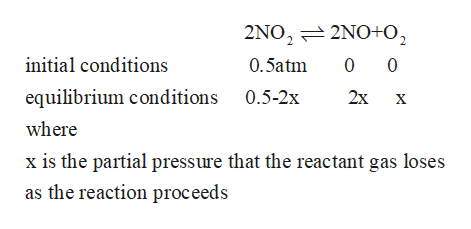# If one starts with pure NO2(g) at a pressure of 0.500 atm, the total pressure inside the reaction vessel when 2NO2(g) <--> 2NO(g) + O2(g) reaches equilibrium is 0.674 atm.  Calculate the equilibrium partial pressure of NO2.

Question

If one starts with pure NO2(g) at a pressure of 0.500 atm, the total pressure inside the reaction vessel when 2NO2(g) <--> 2NO(g) + O2(g) reaches equilibrium is 0.674 atm.  Calculate the equilibrium partial pressure of NO2.

check_circle

Step 1

Given

The NO2 has initial partial pressure = 0.500atm

The reaction that is taking place

Step 2

The conditions as the reaction proceedshelp_outlineImage Transcriptionclose2NO2 2NO+O2 0 0 initial conditions 0.5atm equilibrium conditions 0.5-2x 2x X where x is the partial pressure that the reactant gas loses as the reaction proceeds fullscreen
Step 3

Since the final partial pressur...

### Want to see the full answer?

See Solution

#### Want to see this answer and more?

Solutions are written by subject experts who are available 24/7. Questions are typically answered within 1 hour.*

See Solution
*Response times may vary by subject and question.
Tagged in

### Chemistry